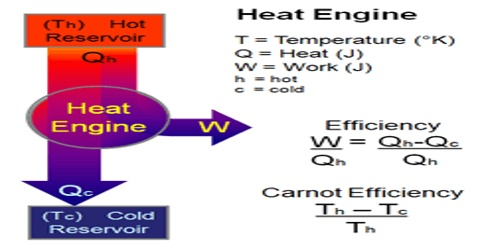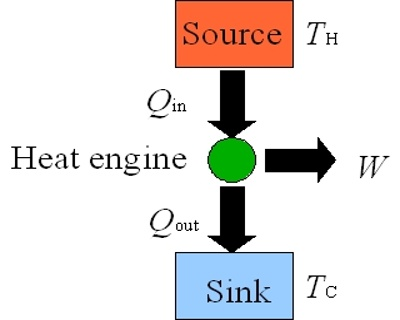# Heat Engine

Heat Engine

Heat engine is a machine which converts thermal energy into work. The discovery of heat engine played a crucial role in many technological developments that include, among others, automobile engines and steam turbines to run generators to produce electricity. A heat engine typically uses energy provided in the form of heat to do work and then exhausts the heat which cannot be used to do work. A schematic diagram of a heat engine is shown in Figure.

In a heat engine heat energy q2 is absorbed from a hot reservoir at temperature T2. The engine does work w (w is negative as work is done by the engine or the system) and discards the remaining heat q1 (q1 is negative as heat is given out) to the cold reservoir at temperature T1. In thermodynamic terms, the hot reservoir is called a ‘Source’ and the cold reservoir is called a ‘Sink’.Figure: schematic diatom of a heat engine

To repeat the process, the engine must be returned to the initial temperature T2 and the steps followed in sequence and we say that it completes a ‘cycle’. However, in order to evaluate the usefulness of a heat engine a quantity, known as ‘Efficiency (E) of the engine, is defined. The efficiency is defined as,

“The ratio of work done to the quantity of energy supplied”.

Mathematically,

E = (Energy out/Energy in) = (Work done/ Energy Supplied)

= (Heat input – Heat discharged) / Heat Supplied

so, E = (qT2 – qT1) / qT2 … … … (1)

From a detailed analysis of the reversible Carnot cycle, Lord Kelvin later concluded that the quantities of heat for a reversible Carnot engine are proportional to absolute temperature. Thus, equation (1) can be written as,

E = (T2 – T1) / T2 … … … (2)

It may, therefore, be concluded that the efficiency of a reversible heat engine is dependent on the temperatures of the source and the sink. High efficiency is obtained when T2 >> T1 and 100% efficiency is predicted when T1 = 0. But in practice 100% efficiency can never be obtained, since there is always some unavailable energy.

Example: What is the efficiency of a reversible cyclic engine operating between temperatures 20°C and 5000C?

Solution: T2 = 273 + 500= 773

and T1 = 273 + 20 = 293

Using equation (2) we get, E = (T2 – T1) / T2

= (773 – 293) / 773 = 0.620